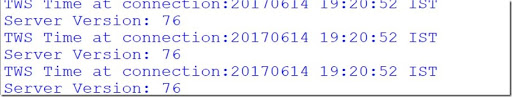### How to Setup Python API Trading Platform.

Hello Everyone,Today I'm here for connecting to TWS with the Help of Python IbPy.

# `````` from ib.opt import Connection,message from ib.ext.Contract import Contract from ib.ext.Order import Order def make_contract(symbol,sec_type,exch,prim_exch,curr): Contract.m_symbol=symbol Contract.m_sec_Type=sec_type Contract.m_exchange=exch Contract.m_primaryExch=prim_exch Contract.m_currency=curr return Contract def make_order(action,quantity,price=None): if price is not None : order=Order() order.m_orderType='LMT' order.m_totalQuantity=quantity order.m_action=action order.m_lmtprice=price return order else: order=Order() order.m_orderType='MKT' order.m_totalQuantity=quantity order.m_action=action return order cid=500 while __name__=="__main__": conn=Connection.create(port=7496,clientId=999) conn.connect() oid=cid cont=make_contract('AAPL','STK','SMART','SMART','USD') offer=make_order('SELL',1,200) conn.placeOrder(oid,cont,offer) conn.disconnect() ````````` ```---End of Code Input--